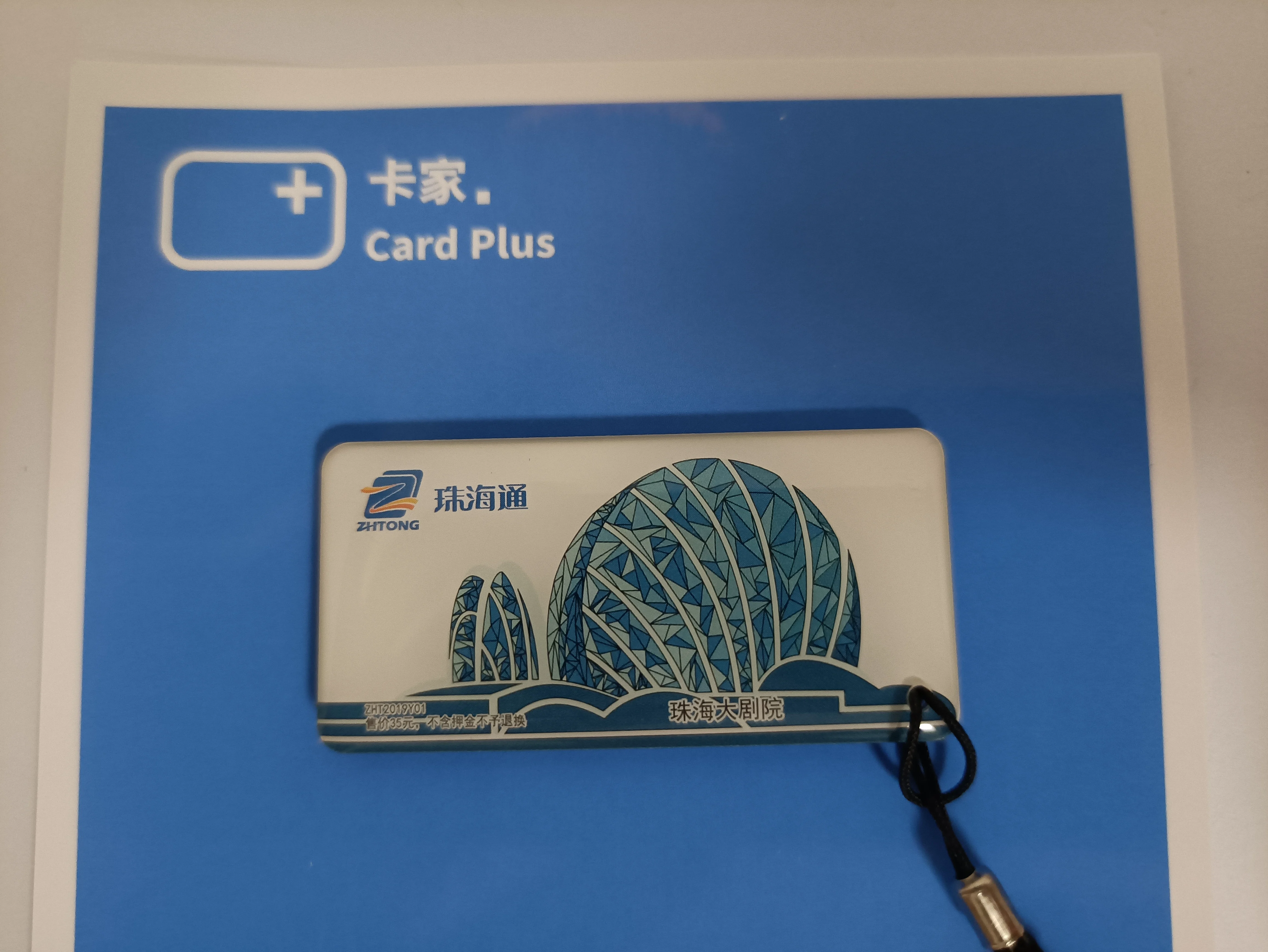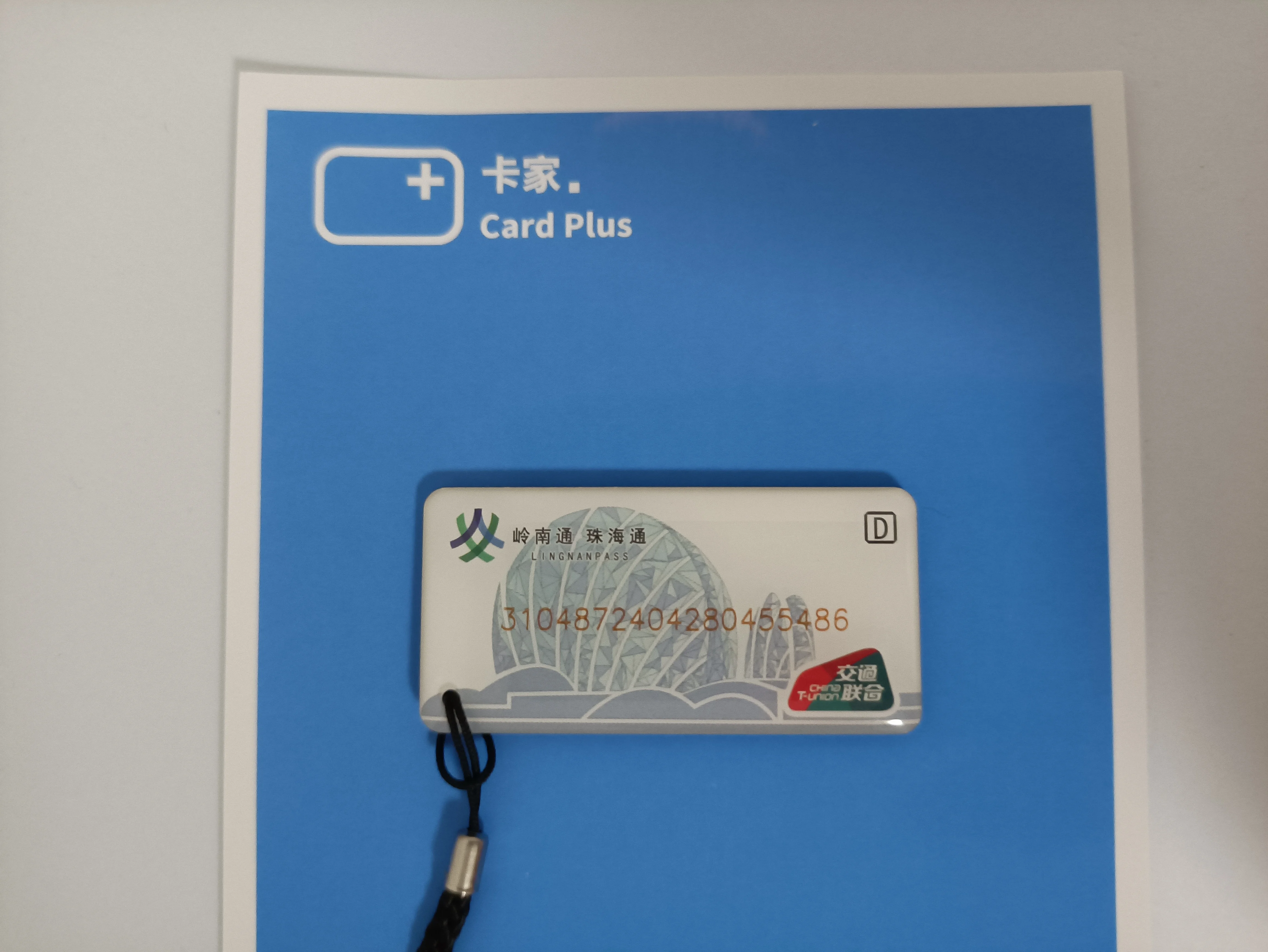3+1+0+4+8+7+2+4+0+4+2+8+0+4+5+5+4+8=69，

加载中...
1. ZBC是不是倒闭了，所以没钱买卡更新Card+？

回复
2. OHL

少吃汉堡多买卡

回复